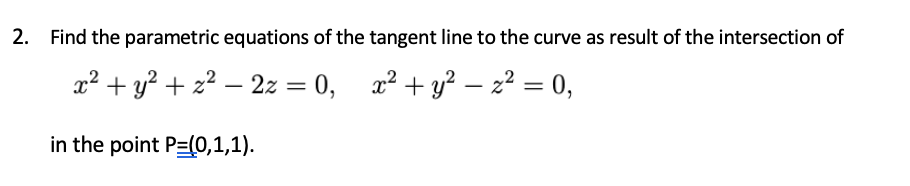# 2. Find the parametric equations of the tangent line to the curve as result of the intersection of x² + y? + z² – 2z = 0, x² + y² – z² = 0, in the point P=(0,1,1).

Questionhelp_outlineImage Transcriptionclose2. Find the parametric equations of the tangent line to the curve as result of the intersection of x² + y? + z² – 2z = 0, x² + y² – z² = 0, in the point P=(0,1,1). fullscreen

### Want to see this answer and more?

Experts are waiting 24/7 to provide step-by-step solutions in as fast as 30 minutes!*

*Response times may vary by subject and question complexity. Median response time is 34 minutes for paid subscribers and may be longer for promotional offers.
Tagged in
Math
Calculus

### Other